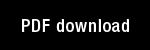Nonlinear difference-differential equations in prediction and learning theory

Author(s): Grossberg, S. |

Year: 1967

Citation: Proceedings of the National Academy of Sciences, 58, 1329-1334

Abstract: Introduction.-This note introduces some nonlinear difference-differential equations which can be interpreted as a learning theory or, alternatively, as a prediction theory whose goal is to discuss the prediction of individual events, in a fixed order, and at prescribed times. The theory provides a mathematical description of the following kind of experiment. An experimenter g, confronted by a machine MlZ, presents M with a list of "letters" or "events" to be learned. Suppose, for example, that g wishes to teach 9Mt the list of letters AB, or to predict the event B, given the event A. g does this by presenting A and then B to M several times. To find out if SE has learned the list as a result of these list presentations, the letter A alone is then presented to M. If M responds with the letter B, and M does this whenever A alone is said, then we have good evidence that MR has indeed learned the list AB. Thus M learns to predict the event B whenever the event A occurs as a result of repeated presentations of the list AB. We will introduce some mathematical machines that...

Topics: Mathematical Foundations of Neural Networks,